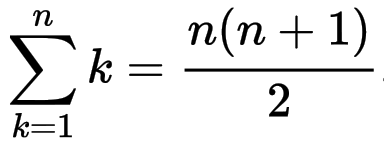# Solution: Sum of numbers from 1 to 100

To compute a sum of integer numbers from 1 to 100, it is, of course, possible to add all those numbers in a single long expression. Or create a loop (we’ll cover loops in the further sections). But the best solution is to use a well-known formula:With the formula, the solution becomes rather trivial.

## Code

```my \$N = 100;
my \$sum = \$N * (1 + \$N) / 2;
say "The sum of the numbers from 1 to \$N is \$sum.";
```

🦋 You can find the full code in the file sum1-100.raku.

## Output

``````\$ raku exercises/numbers/sum1-100.raku
The sum of the numbers from 1 to 100 is 5050.
``````